• ## 项目反应理论

千次阅读 2018-01-07 11:58:59
项目反应理论（item response theory）是属于心理学中认知诊断常用的一种理论，即根据被测试者针对某个问题的答案来对被测者的认知状况进行估计。“项目”实质就是测试题，“反应”就是被测者的答案。也有学者称项目...
项目反应理论（item response theory）是属于心理学中认知诊断常用的一种理论，即根据被测试者针对某个问题的答案来对被测者的认知状况进行估计。“项目”实质就是测试题，“反应”就是被测者的答案。也有学者称项目反应理论也是机器学习中的一个类别，即根据被测者对测试题的反应来判定被测者所属的类别。

1、 项目特征曲线

项目特征曲线可用来描述项目（问题）难度与区分度，即项目的难度与区分度是项目的两个维度。下图展示了一个典型的项目（问题）特征曲线。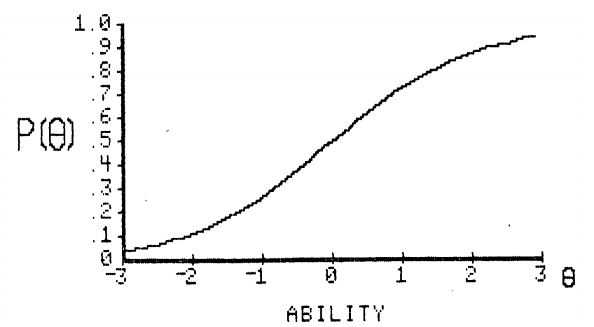图中，横坐标表示能力值，纵坐标表示做出该题的概率。显然，能力值越低做出该题的概率越小，能力值越高做出该题的概率越大。

一个项目的难度可以分为[很容易，容易，中等，难，很难]五个层次
一个项目的区分度可以分为[很低，低，中等，高，很高]五个层次
因此，每一个项目都有25种可能的曲线

上图中的项目特征曲线表示中等的难度与中等的区分度，类似地还有中等难度高区分度的曲线等等，详见item response theory。

2、项目特征曲线模型

如何用一个数学表达式来表示项目特征曲线是项目特征曲线建模需要考虑的问题。目前主要有三种模型，这三种模型本质上都属于Logistic。

2.1 Logistic模型

P(θ)=11+e−a(θ−b)<!--//--><![CDATA[//><!--
P(\theta)=\frac{1}{1+e^{-a(\theta-b)}}
//--><!]]>

b：代表项目难度系数，理论上可以取（-∞<!--//--><![CDATA[//><!--
\infty
//--><!]]>,+∞<!--//--><![CDATA[//><!--
\infty
//--><!]]>）,典型值在[-3,3]之间
a：代表项目区分度系数，理论上可以取（-∞<!--//--><![CDATA[//><!--
\infty
//--><!]]>,+∞<!--//--><![CDATA[//><!--
\infty
//--><!]]>），典型值在[-2.8,2.8]之间
θ<!--//--><![CDATA[//><!--
\theta
//--><!]]>：代表能力值
2.2 Rasch模型

Rasch是Logistic模型的一种情况，也称为一个参数的Logistic模型。当Logistic模型中项目区分度a的值为1时，即为Rasch模型。

P(θ)=11+e−1(θ−b)<!--//--><![CDATA[//><!--
P(\theta)=\frac{1}{1+e^{-1(\theta-b)}}
//--><!]]>

b：代表项目难度系数，理论上可以取（-∞<!--//--><![CDATA[//><!--
\infty
//--><!]]>,+∞<!--//--><![CDATA[//><!--
\infty
//--><!]]>）,典型值在[-3,3]之间
θ<!--//--><![CDATA[//><!--
\theta
//--><!]]>：代表能力值
2.3 三参数模型

P(θ)=c+1−c1+e−a(θ−b)<!--//--><![CDATA[//><!--
P(\theta)=c+\frac{1-c}{1+e^{-a(\theta-b)}}
//--><!]]>

b：代表项目难度系数，理论上可以取（-∞<!--//--><![CDATA[//><!--
\infty
//--><!]]>,+∞<!--//--><![CDATA[//><!--
\infty
//--><!]]>）,典型值在[-3,3]之间
a：代表项目区分度系数，理论上可以取（-∞<!--//--><![CDATA[//><!--
\infty
//--><!]]>,+∞<!--//--><![CDATA[//><!--
\infty
//--><!]]>），典型值在[-2.8,2.8]之间
c：代表猜测参数，猜测参数取值范围是[0,1]，典型值通常超过0.35
θ<!--//--><![CDATA[//><!--
\theta
//--><!]]>：代表能力值
注意：猜测系数与个人能力值无关，也就是高能力与低能力值的被测试者有相同的概率通过猜测答对题目。
展开全文机器学习
• 项目反应理论参数的EM估计 IRT（项目反应理论）广泛应应用于心理测量学，相比CTT（传统测量理论）的主要优势在于不依赖于样本，能够为被试者提供具有一致性的测量结果。 IRT的基本假设： 单维性，即能力是单维度的...
项目反应理论参数的EM估计
写在前面：
本文主要描述了整个IRT使用EM算法参数的估计过程，其中涉及大量公式，如只是需要了解IRT相关基础知识，请转战wiki～～
预警： 大量公式来袭～～


IRT（项目反应理论）广泛应应用于心理测量学，相比CTT（传统测量理论）的主要优势在于不依赖于样本，能够为被试者提供具有一致性的测量结果。
IRT的基本假设：

单维性，即能力是单维度的。
局部独立性， 即项目间局部独立。
项目反应函数假设，即被试者对项目对反应符合项目反应方程。

IRT 模型：
常见的IRT模型包括2PL模型和3PL模型，其中2PL模型表达式如下：$p _ { i , j } \left( \theta _ { i } \right) = \frac { 1 } { 1 + \exp \left[ - D a _ { j } \left( \theta _ { i } - b _ { j } \right) \right] }$
其中$\theta _ { i }$表示被试者的能力，$a _ { j }$是项目的区分度，$b _ { j }$是项目的难度，D=1.7。
参数估计：
对于IRT参数估计有多种方法，如对于项目参数的估计有：边际极大似然估计（MMLE），极大边际后验法（MMAP），EM，MCMC等方法，对于能力参数的估计有极大似然（MLE），贝叶斯众数法（MAP），贝叶斯后验期望估计法（EAP）等。本文重点介绍对项目参数的EM估计。
建模：

被观测数据$\mathbf { Y }$是N*J维的，N表示有N个被试者，J表示有J个题目，$\mathbf { Y } = \left( \mathbf { y } _ { 1 } , \mathbf { y } _ { 2 } , \dots , \mathbf { y } _ { N } \right)$，$\mathbf { y } _ { i }=\left( y _ { i 1 } , y _ { i 2 } , \ldots , y _ { i J } \right)$，其中$y _ { i j }$表示第i个被试者回答第j道题目的答案；
不可观测变量为$\boldsymbol { \theta } = \left( \theta _ { 1 } , \theta _ { 2 } , \ldots , \theta _ { N } \right)$ 其中$\theta _ { i }$是第i个被试者的能力值，模型中假定$\theta _ { i }$只能取离散的值$q _ { k } , k = 1 , \dots , K$，对应取$q _ { k }$的概率为$\pi _ { k } , k = 1 , \ldots , K$，即$\theta _ { i }$为多项式分布，其概率为：$\boldsymbol {\pi} = \left( \pi _ { 1 } , \pi _ { 2 } , \dots , \pi _ { K } \right)$；不可观测变量为$\boldsymbol { \theta } = \left( \theta _ { 1 } , \theta _ { 2 } , \ldots , \theta _ { N } \right)$ 其中$\theta _ { i }$是第i个被试者的能力值，模型中假定$\theta _ { i }$只能取离散的值$q _ { k } , k = 1 , \dots , K$，对应取$q _ { k }$的概率为$\pi _ { k } , k = 1 , \ldots , K$，即$\theta _ { i }$为多项式分布，其概率为：$\boldsymbol {\pi} = \left( \pi _ { 1 } , \pi _ { 2 } , \dots , \pi _ { K } \right)$；
完全数据为$\left[ \left( \mathbf { y } _ { 1 } , \theta _ { 1 } \right) , \left( \mathbf { y } _ { 2 } , \theta _ { 2 } \right) , \ldots , \left( \mathbf { y } _ { N } , \theta _ { N } \right) \right]$；完全数据为$\left[ \left( \mathbf { y } _ { 1 } , \theta _ { 1 } \right) , \left( \mathbf { y } _ { 2 } , \theta _ { 2 } \right) , \ldots , \left( \mathbf { y } _ { N } , \theta _ { N } \right) \right]$；
待估计参数为项目参数$\boldsymbol {\Delta}=(\boldsymbol { \delta } _ { 1 },\boldsymbol { \delta } _ { 2 },...\boldsymbol { \delta } _ { j })$，其中$\boldsymbol { \delta } _ { j }$为第j道题的参数。待估计参数为项目参数$\boldsymbol {\Delta}=(\boldsymbol { \delta } _ { 1 },\boldsymbol { \delta } _ { 2 },...\boldsymbol { \delta } _ { j })$，其中$\boldsymbol { \delta } _ { j }$为第j道题的参数。

EM算法应用于IRT中是迭代估计$\boldsymbol {\Delta}$ 和$\boldsymbol {\pi}$:
E step:  根据给定的缺失数据的分布，观察数据和参数初始值，求完全数据的对数似然函数的条件期望。
M step:   极大化E-step给出的完全数据的对数似然函数的条件期望，求参数的值。
似然函数
给定观测变量$\mathbf { y } = \left( y _ { 1 } , y _ { 2 } , \ldots , y _ { J } \right)$并假定能力参数分布为：$\pi = \left( \pi _ { 1 } , \pi _ { 2 } , \dots , \pi _ { J } \right)$，项目参数为$\boldsymbol {\Delta}=(\boldsymbol { \delta } _ { 1 },\boldsymbol { \delta } _ { 2 },...\boldsymbol { \delta } _ { j })$，则观测变量的条件概率分布为：$f ( \mathbf { y } | \mathbf { \Delta } , \boldsymbol { \pi } ) = \sum _ { k = 1 } ^ { K } f \left( \mathbf { y } , q _ { k } | \Delta , \pi _ { k } \right)$$= \sum _ { k = 1 } ^ { K } f ( \mathbf { y } | q _ { k } , \Delta ) \pi _ { k }$
由IRT的局部独立性假设，由于给定能力变量下项目反应结果是相互独立的，可得：$f ( \mathbf { y } | q _ { k } , \mathbf { \Delta } ) = \prod _ { j = 1 } ^ { J } P \left( q _ { k } | \boldsymbol { \delta } _ { j } \right) ^ { y _ { j } } \left[ 1 - P \left( q _ { k } | \boldsymbol { \delta } _ { j } \right) \right] ^ { 1 - y _ { j } }$
其中$P \left( q _ { k } | \boldsymbol { \delta } _ { j } \right)$是题目j的项目特征曲线，描述了给定能力下项目j答对的概率。由上式可以推出，完全数据的似然函数为：\begin{aligned} L ( \mathbf { Y } , \boldsymbol { \theta } | \mathbf { \Delta } , \boldsymbol { \pi } ) & = \prod _ { i = 1 } ^ { N } \prod _ { j = 1 } ^ { J } P \left( \theta _ { i } | \boldsymbol { \delta } _ { j } \right) ^ { y _ { i j } } \left[ 1 - P \left( \theta _ { i } | \boldsymbol { \delta } _ { j } \right) \right] ^ { 1 - y _ { i j } } f \left( \theta _ { i } | \boldsymbol { \pi } \right) \\ & = \prod _ { j = 1 } ^ { J } \prod _ { i = 1 } ^ { N } P \left( \theta _ { i } | \boldsymbol { \delta } _ { j } \right) ^ { y _ { i j } } \left[ 1 - P \left( \theta _ { i } | \boldsymbol { \delta } _ { j } \right) \right] ^ { 1 - y _ { i j } } f \left( \theta _ { i } | \boldsymbol { \pi } \right) \\ & = \prod _ { j = 1 } ^ { J } \prod _ { k = 1 } ^ { K } P \left( \theta _ { k } | \boldsymbol { \delta } _ { j } \right) ^ { r _ { j k } } \left[ 1 - P \left( q _ { k } | \boldsymbol { \delta } _ { j } \right) \right] ^ { n _ { k } - r _ { j k } } \pi _ { k } ^ { n _ { k } } \end{aligned}
其中，$f \left( \theta _ { i } | \boldsymbol { \pi } \right) = \pi _ { k }$ if $\theta _ { i } = q _ { k }$，$n _ { k }$是N个被试者中能力为$q _ { k }$的人数，$\boldsymbol { r }_{ j k}$则是$n _ { k }$人中答题正确的人数。
为方便计算，将上式化成对数似然函数为：$\log [ L ( \mathbf { R } , \mathbf { n } | \mathbf { \Delta } , \boldsymbol { \pi } ) ] = \sum _ { j = 1 } ^ { J } \sum _ { k = 1 } ^ { K } r _ { j k } \log \left[ P \left( q _ { k } | \boldsymbol { \delta } _ { j } \right) \right] + \left( n _ { k } - r _ { j k } \right) \log \left[ 1 - P \left( q _ { k } | \boldsymbol { \delta } _ { j } \right) \right] + n _ { k } \log \left[ \pi _ { k } \right]$
E-Step:
E步估计隐变量$\boldsymbol { \theta}$，在给定项目参数$\boldsymbol {\Delta}^{(s)}$ 和能力分布$\boldsymbol {\pi}^{(s)}$(由M步迭代估计得到)的和观测变量下隐变量的条件分布为：
\begin{aligned} f \left( q _ { k } | \mathbf { y } _ { i } , \Delta ^ { ( s ) } , \pi ^ { ( s ) } \right) & = \frac { f \left( \mathbf { y } _ { i } | q _ { k } , \mathbf { \Delta } ^ { ( s ) } \right) \pi _ { k } ^ { ( s ) } } { \sum _ { k ^ { \prime } = 1 } ^ { K } f \left( \mathbf { y } _ { i } | q _ { k ^ { \prime } } , \Delta ^ { ( s ) } \right) \pi _ { k ^ { \prime } } ^ { ( s ) } } \\ & = \frac { \pi _ { k } ^ { ( s ) } \prod _ { j = 1 } ^ { J } P \left( q _ { k } | \boldsymbol { \delta } _ { j } ^ { ( s ) } \right) ^ { y _ { i j } } \left[ 1 - P \left( q _ { k } | \boldsymbol { \delta } _ { j } ^ { ( s ) } \right) \right] ^ { 1 - y _ { i j } } } { \sum _ { k ^ { \prime } = 1 } ^ { K } \pi _ { k ^ { \prime } } ^ { ( s ) } \prod _ { j = 1 } ^ { J } P \left( q _ { k ^ { \prime } } | \boldsymbol { \delta } _ { j } ^ { ( s ) } \right) ^ { y _ { i j } } \left[ 1 - P \left( q _ { k ^ { \prime } } | \boldsymbol { \delta } _ { j } ^ { ( s ) } \right) \right] ^ { 1 - y _ { i j } } } \end{aligned}
$n _ { k } ^ { ( s ) } = E \left( n _ { k } | \mathbf { Y } , \Delta ^ { ( s ) } , \boldsymbol { \pi } ^ { ( s ) } \right)= \sum _ { i = 1 } ^ { N } f \left( q _ { k } | \mathbf { y } _ { i } , \mathbf { \Delta } ^ { ( s ) } , \boldsymbol { \pi } ^ { ( s ) } \right)= \sum _ { i = 1 } ^ { N } \frac { f \left( \mathbf { y } _ { i } | q _ { k } , \mathbf { \Delta } ^ { ( s ) } \right) \pi _ { k } ^ { ( s ) } } { \sum _ { k ^ { \prime } = 1 } ^ { K } f \left( \mathbf { y } _ { i } | q _ { k ^ { \prime } } , \boldsymbol { \Delta } ^ { ( s ) } \right) \pi _ { k ^ { \prime } } ^ { ( s ) } }= \sum _ { i = 1 } ^ { N } \frac { \pi _ { k } ^ { ( s ) } \prod _ { j = 1 } ^ { J } P \left( q _ { k } | \boldsymbol { \delta } _ { j } ^ { ( s ) } \right) ^ { y _ { i j } } \left[ 1 - P \left( q _ { k } | \boldsymbol { \delta } _ { j } ^ { ( s ) } \right) \right] ^ { 1 - y _ { i j } } } { \sum _ { k ^ { \prime } = 1 } ^ { K } \pi _ { k ^ { \prime } } ^ { ( s ) } \prod _ { j = 1 } ^ { J } P \left( q _ { k ^ { \prime } } | \boldsymbol { \delta } _ { j } ^ { ( s ) } \right) ^ { y _ { i j } } \left[ 1 - P \left( q _ { k ^ { \prime } } | \boldsymbol { \delta } _ { j } ^ { ( s ) } \right) \right] ^ { 1 - y _ { i j } } }$
$r _ { j k } ^ { ( s ) } = {y}_{ij}n _ { k } ^ { ( s ) }$
M-Step:
M步是使用E步计算的$n _ { k } ^ { ( s ) }$和$r _ { j k } ^ { ( s ) }$去迭代估计$\Delta , \pi$。根据上述的对数似然函数可得：$\log \left[ L \left( \mathbf { R } ^ { ( s ) } , \mathbf { n } ^ { ( s ) } | \Delta , \boldsymbol { \pi } \right) \right] = \sum _ { j = 1 } ^ { J } l \left( \boldsymbol { \delta } _ { j } \right) + l ( \boldsymbol { \pi } )$
其中$l \left( \boldsymbol { \delta } _ { j } \right) = \sum _ { k = 1 } ^ { K } r _ { j k } ^ { ( s ) } \log \left[ P \left( q _ { k } | \boldsymbol { \delta } _ { j } \right) \right] + \left( n _ { k } ^ { ( s ) } - r _ { j k } ^ { ( s ) } \right) \log \left[ 1 - P \left( q _ { k } | \boldsymbol { \delta } _ { j } \right) \right]$
$l ( \boldsymbol { \pi } ) = \sum _ { k = 1 } ^ { K } n _ { k } ^ { ( s ) } \log \left[ \pi _ { k } \right]$
使用极大似然估计求$\Delta , \pi$，由于$\pi$是服从多项式分布的，所以很容易得到$\pi _ { k } ^ { ( s + 1 ) } = \frac { n _ { k } ^ { ( s ) } } { N }$
为求$\Delta$，对$l \left( \boldsymbol { \delta } _ { j } \right)$关于$\boldsymbol { \delta } _ { j }$求导：$\frac { \partial l \left( \boldsymbol { \delta } _ { j } \right) } { \partial \delta _ { t j } } = 0$
$\sum _ { k = 1 } ^ { K } \frac { r _ { j k } ^ { ( s ) } - n _ { k } ^ { ( s ) } P \left( q _ { k } | \boldsymbol { \delta } _ { j } \right) } { \left[ 1 - P \left( q _ { k } | \boldsymbol { \delta } _ { j } \right) \right] P \left( q _ { k } | \boldsymbol { \delta } _ { j } \right) } \frac { \partial P \left( q _ { k } | \boldsymbol { \delta } _ { j } \right) } { \partial \delta _ { t j } } = 0$
结果：
使用12000名学生的约100万条做题记录进行IRT参数估计，其中涉及题目约1000道左右，首先使用EM算法对题目参数进行估计，然后使用EAP对学生能力进行估计，结果分别如下：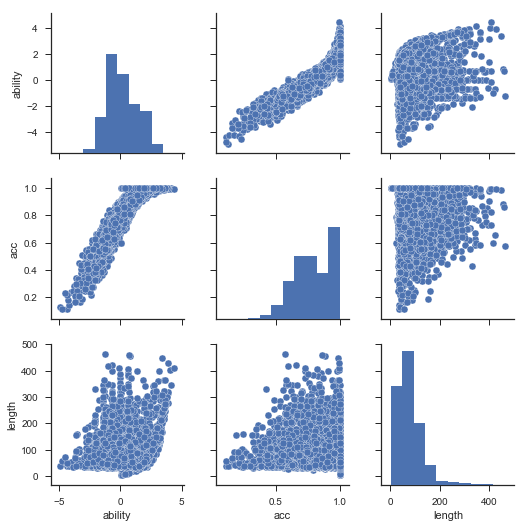上图中，ability为学生能力对评估结果，acc为学生实际答题的准确率，length为做题记录的长度，从图中可以看出，估计出的学生能力与实际答题的准确率正相关，且估计出的学生能力分布呈正态。相同做题准确率下，不同做题长度对评估结果也有影响。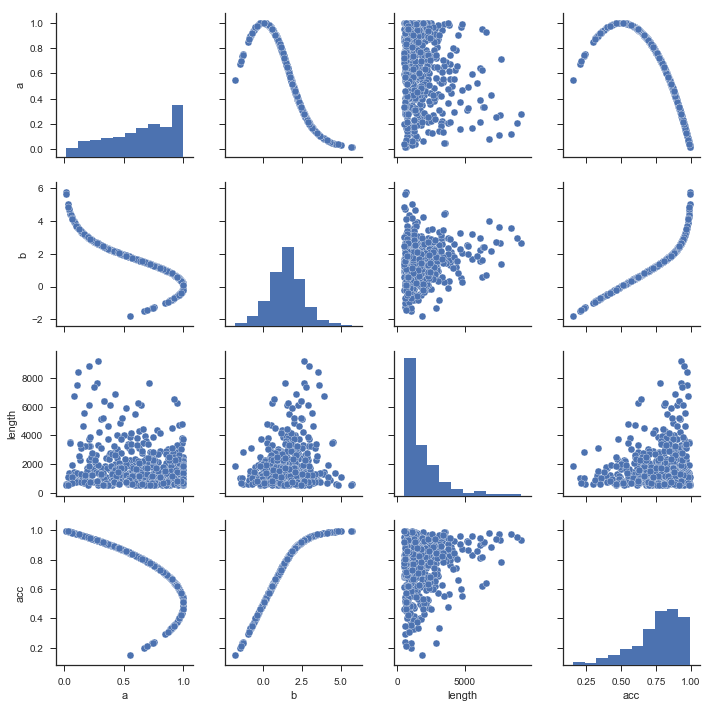上图为估计的题目参数，a表示题目的区分度，b表示题目的难度，可以看出题目难度和题目的准确率严格正相关，区分度和准确率呈倒U型。

参考文献：
1. http://www.openirt.com/b-a-h/papers/note9801.pdf IRT Parameter Estimation using the EM Algorithm
2. https://zhuanlan.zhihu.com/p/29887184 Python与项目反应理论：基于EM和MCMC的参数估计算法
. https://www.cnblogs.com/jiangxinyang/p/9621997.html IRT模型的参数估计方法（EM算法和MCMC算法）
. https://www.john-uebersax.com/stat/irt2.htm How to Calculate Expected A Posteriori (EAP) Scores for Unidimensional and Multidimensional Latent Trait Models
. https://en.wikipedia.org/wiki/Item_response_theory  Item response theory


展开全文•研究论文
• 为了提供一个高效、准确的HSK分级考试系统，文中提出了一种基于项目反应理论的对外汉语教学（HSK）自适应分级考试系统。该系统基于项目反映理论，并以试题作为一个基本的测试项目。当测试开始时，测试者可以自选等级...
• python实现的自适应cat的数学模型-项目反应理论 1.初始能力值确定 2.最大信息量计算 3.解极大似然函数-牛顿迭代法
• 项目反应理论（IRT item response theory） 概述 历史发展 特点 模型 A.项目反应理论（IRT item response theory） 概述 IRT理论即项目反应理论(Item Response Theory, IRT)，又称题目反应理论、潜在特质...
目录

A.项目反应理论（IRT item response theory）

概述

历史发展

特点

模型

A.项目反应理论（IRT item response theory）

概述

IRT理论即项目反应理论(Item Response Theory, IRT)，又称题目反应理论、潜在特质理论（Item Response Theory）是一系列心理统计学模型的总称。IRT是用来分析考试成绩或者问卷调查数据的数学模型。这些模型的目标是来确定的潜在心理特征(latent trait）是否可以通过测试题被反应出来，以及测试题和被测试者之间的互动关系。目前广泛应用在心理和教育测量领域。
项目反应理论的意义在于可以指导项目筛选和测验编制。项目反应理论假设被试有一种“潜在特质”，潜在特质是在观察分析测验反应基础上提出的一种统计构想，在测验中，潜在特质一般是指潜在的能力，并经常用测验总分作为这种潜力的估算。项目反应理论认为被试在测验项目的反应和成绩与他们的潜在特质有特殊的关系。通过项目反应理论建立的项目参数具有恒久性的特点，意味着不同测量量表的分数可以统一。项目反应理论通过项目反应曲线综合各种项目分析的资料，使我们综合直观地看出项目难度、鉴别度等项目分析的特征，从而起到指导项目筛选和编制测验比较分数等作用

历史发展

IRT理论发端于20世纪50年代，它同时被丹麦统计学家Georg Rasch和美国心理统计学家Frederic M. Lord在各自的国家发展起来。尽管采取的研究方法不同，但是他们的结果却非常相似。
F. Lord在1951年从普林斯顿大学毕业时的博士论文《A Theory of Test Scores》被认为是IRT理论的开端之作。在随后的30年中他进入ETS工作不断深入研究这个问题并且在1980年出版的《Applications of Item Response Theory to Practical Testing Problems》正式完善了整个IRT理论的框架。
和Lord几乎在同时，G. Rasch在丹麦政府的委托之下开始研究现代考试理论，他采用了和Lord截然不同的切入点，一开始的时候他称之为潜在特征模型(latent trait model)，却发现了极为类似的结果

特点

(1)独立性。 被试特质水平不依赖于被试样本的代表性；被试水平参数不依赖于测验项目组；项目特征参数不依赖于所测被试组的参数不变测验项目组。
(2)项目理论中被试水平和项目难度可以直接比较；

(3)正视了测量误差和项目性能是否与被试水平相关这一事实；

(4)提供了计算机化自适应测验这一策略；

(5) 从计量学角度提出了自己的新观点与新技术。

模型

模型是用于评估被试对某一项目或某一类项目的潜在特质。
IRT模型是建立在一定的假设之下：

单维性假设，即假设某个测验只测量被试的某一种能力。

立性假设，即假设被试在每一个项目上的作答反应是相互独立，互不影响的，作答反应只与被试自身的能力水平有关，与其他元素无关。

模型假设，即被试在项目上的正确反应概率与被试的能力水平有一定的函数关系。

IRT有一参数、两参数、三参数模型，三参数模型的数学公式如下：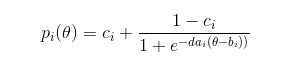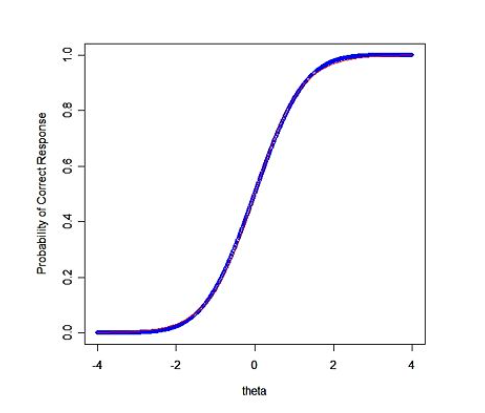根据这模型所绘制出的曲线也叫做项目特征曲线（Item Characteristic Curve, ICC）。其意义在于描述出“成功解答某一特定考试项目的可能性”和“被测试者能力”（在函数中以θ表示）之间的关系。

参数意义：参数c一般被称为“猜测参数”（guessing parameter）或者“伪猜测参数”(pseudo-guessing parameter）。在图像上，c所代表的是ICC的下限，其直观意义为：当一个被测试者的能力值非常低（比如接近负无穷），但是他仍然能够有可能做对这道题目的概率c就是他猜测的能力。b叫做项目难度参数，也称为项目难度(item difficulty)。b一般表示在ICC图像最陡的那一点所对应的θ值。对于下限为0的ICC函数来说，b所对应的是概率为0.5的测试者能力值。改变b会导致ICC的左右移动，但是不改变其形状。当b值增加，会使ICC曲线向右移动（θ值高的的方向），这会引起在即使θ保持不变，但是答题正确率下降，亦即题目难度增加。反之当b值减小，ICC曲线向左移动。题目难度降低。a叫做区分度参数或项目区分度(item discrimination)。在数学上，a的值是ICC曲线拐点处的斜率，即斜率的最大值。在这一点上，能力值微小的改变会造成最大的P值（回答正确率）变动。所以a体现的是该项目的最大区分度。

IRT简化的一参数模型Rasch模型：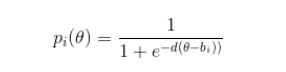在Rasch模型中，所有的曲线，其形状都是一样的。实际上，这是不合理的。比如，有两道难度相同(比如难度等于2)的题目，一道是判断题，一道是选择题(4个选项)。对于判断题，即使学渣完全不知道怎么做，也有大约有50%概率能答对，而学霸也是50%左右的概率能答对。对于选择题，学渣大约有25%概率能答对，而学霸仍然是50%左右。因此，选择题比判断题能够更好的区分学霸和学渣，我们称它的区分度(discrimination)更高。本次实验的数据来自于学生oj题目数据，所有题目都属于同一种类型，因此不需要引入区分度来进行区别。与此同时，蒙对一道oj题目的概率较小，因此猜测系数在本次实验中也不考虑。因此采用Rasch模型进行学生oj做题情况预测。参数估计 IRT模型的参数估计方法有很多，包括极大似然估计，EM算法，贝叶斯算法等，本文将简单介绍使用极大似然估计方法进行参数估计过程。

在知识追踪方面：IRT对单个技能进行建模，并假设测试项目是一维的。它为学生i分配静态能力θi。 每个项目j都有自己的难度βj。 IRT的主要思想是利用学生的能力和难度来估计学生i正确回答项目j的可能性。 广泛使用的IRT一个参数版本（称为Rasch模型）是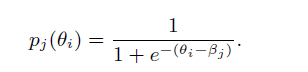最近，Wilson 提出了一种IRT模型，该模型优于最新的知识跟踪模型。 其中，使用牛顿-拉夫森方法计算θi和βj的最大后验（MAP）估计。

实验部分详见博客：

参考资料

论文：Deep Knowledge Tracing and Dynamic Student Classification for Knowledge Tracing

博客：https://blog.csdn.net/qq_40275208/article/details/104202061

https://wiki.mbalib.com/wiki/IRT理论

展开全文• 92年的项目反应理论基础用书，详细给出了基础模型
• 项目反应理论—–部分介绍了IRT理论 百度百科介绍项目反应理论—较详细 IRT即项目反应理论发展于1950s到1960s。在IRT理论中，被试者回答问题或者项目的正确与否依赖于线性连续潜在特征θ\theta，也就是被测者的能力...
IRT即项目反应理论发展于1950s到1960s。

在IRT理论中，被试者回答问题或者项目的正确与否依赖于线性连续潜在特征θ<!--//--><![CDATA[//><!--
\theta
//--><!]]>，也就是被测者的能力。
局部独立性假设：给定潜在特征θ<!--//--><![CDATA[//><!--
\theta
//--><!]]>，项目的回答被认为是条件独立的并且遵循某种分布p(θ)=Pr(Xj=1|θ)<!--//--><![CDATA[//><!--
p(\theta)=Pr(X_j=1|\theta)
//--><!]]>

函数p(θ)<!--//--><![CDATA[//><!--
p(\theta)
//--><!]]>叫做项目反应函数，项目特征曲线或者是项目曲线。
它一般随着θ<!--//--><![CDATA[//><!--
\theta
//--><!]]>的增加单调递增，所以被测者能力水平越高，那么回答对项目的概率越高。

Rasch model:一个单参数logistic IRT模型。
对于第i个项目的项目反应函数是：

pi(θ)=11+exp(−(θ−bi))<!--//--><![CDATA[//><!--
p_i(\theta)=\frac{1}{1+exp(-(\theta-b_i))}
//--><!]]>
其中bi<!--//--><![CDATA[//><!--
b_i
//--><!]]>表达项目的难度。

需要强假设，假定所有的项目都以类似的方式进行区分。

Birnbaum’s two parameter logistic model(2-PL)

对于第i个项目的项目反应函数是：

pi(θ)=11+exp(−ai(θ−bi))<!--//--><![CDATA[//><!--
p_i(\theta)=\frac{1}{1+exp(-a_i(\theta-b_i))}
//--><!]]>
其中bi<!--//--><![CDATA[//><!--
b_i
//--><!]]>表达项目的难度。
其中ai<!--//--><![CDATA[//><!--
a_i
//--><!]]>表示项目区分参数，它的值越大说明题目对受测者的区分程度越高

参考资料：

项目反应理论—–部分介绍了IRT理论
百度百科介绍项目反应理论—较详细

展开全文• 探讨了几种常用的基于项目反应理论(I RT) 的试题参数估计方法, 并分析了每一种估计方法的优缺点及各自的适用领域, 为构建基于IRT的试题库系统提供理论参考。
• 我们基于项目响应理论提出了一个模型，该模型突出了潜在特征（称为“熟练度”和“倾向性”）的作用。 对该模型进行了调整，以适应来自10,822名巴西高中学生参加的高分考试所做出决定的数据。 我们的模型旨在恢复有关...
• 项目反应理论的开端早在上世纪初，智力测验的发明者比奈(也可能是西蒙)便发现了一条神奇的曲线，这条曲线的x轴是智力水平，y轴是试题正确率，而这是项目反应理论(以下简称IRT)的最初雏形。上世界五六十年代，ETS的...
• vipsyvipsy是《变分推断在教育测量模型中的...斯坦福大学项目存在的问题：多维项目反应模型的参数估计精度严重失真，乃至不收敛，该项目只适合单维项目反应理论。重参数方法不适用于认知诊断模型，不具备普适性本...
• 状态是给定类的对象的一组属性值 ,这组属性值对所发生的事件具有相同性质的反应。状态机用于描述类的行为，但它们也描述用例、协作和方法的动态行为。 2. 状态机 状态机是展示状态与状态转换的图。通常一个...
• 项目反应理论与经典测验理论之比较 经典测验理论简称为CTT，假定个人经过测验的真实得分为T，这个得分是在没有测量误差的情况下得到的，观察分数X是在加入了额外的误差E后得到的。 也就是说X=T+EX=T+E信度的定义：...
• 前段时间，公司的项目从PHP5.3升级到PHP7，现在项目里开始使用PHP7的一些新语法和特性。反观PHP的5.4、5.5、5.6版本，有点认知缺失的感觉。所以，决定看《Modern PHP》补一补里面的一些概念。一、命名空间命名空间用...
• 这系列相关博客，参考 设计模式之美 设计模式之美 - 100 | 如何将设计思想、原则、模式...实际上，很多小伙伴反应，虽然理论掌握得差不多，专栏也很贴近实战，每个知识点的讲解都有结合实际的代码案例，并且最后还有集java 设计模式
• multilog7.03软件，供项目反应理论者使用，可以用于单参数或多参数分析。
• 自编心理测量库，包含多维项目反应理论，认知诊断，因子分析和自适应测验等等
• 结合经典测量理论（CTT）和项目反应理论（IRT）的优点，建立了计算机自适应题库。本题库能为应试者建立更适合自己能力的个性化测验，在评估应试者真实能力方面取得了良好的效果。
• 离线数据仓库项目上数据仓库理论数据仓库什么是数据仓库数据仓库四大特征数据仓库作用数据仓库与数据库的区别数据集市数据模型数据仓库分层数据仓库模型元数据数据仓库命名规范实践 用户活跃度分析需求分析日志数据...数据仓库
• 多维IRT模型的EM估计 MIRT （Multidimensional Item Response Theory）多维项目反应理论。模型如下，EM算法 梯度下降
• 计算机自适应测验中RASCH模型稳健性的模拟研究，邓远平，罗照盛，计算机自适应测验（Computerized Adaptive Test，以下简称CAT）是建立在项目反应理论（Item Response Theory）的基础上，按照一定的策略进行选题�
• 认知诊断测验的属性分类一致性和分类准确性指标，汪文义，宋丽红，分类一致性和分类准确性是衡量信效度的两个重要的考试质量评价指标。基于项目反应理论下分类一致性和分类准确性指标，提出认知诊
• ## IRT模型估计-EM算法

千次阅读 2019-05-22 09:12:28
IRT(Item Response Theory) 项目反应理论。是教育评估与心理测量理论中的重要模型。主要目的是通过被试(examinees) 的对于一套试题的反应(responses), 对被试 的能力(ability parameters) 参数θ\thetaθ 和题目...em算法...